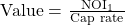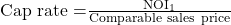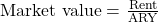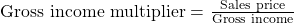Essential Concept 82: Income Approach to Value Real Estate | IFT World
101 Concepts for the Level I Exam

# Essential Concept 82: Income Approach to Value Real EstateThe income approach focuses on net operating income generated from a property. NOI is roughly analogous to EBITDA.  We can calculate NOI as shown below:

Rental income at full occupancy

+ Other income

= Potential gross income

– Vacancy and collection loss

= Effective gross income

– Operating expenses

= Net operating income

The income approach includes two different valuation methods: direct capitalization method and discounted cash flow method.

Direct capitalization method:

Value is based on capitalizing the first year NOI of the property using a cap rate.If the NOI and value of a property are expected to grow at a constant rate, then the cap rate is given by:

Cap rate = Discount rate – Growth rate

Cap rate can also be estimated from comparables.When tenants are required to pay all expenses, a cap rate may be applied to rent instead of NOI. The cap rate that is obtained by dividing rent by comparable sales is called the ‘all risks yield’ (ARY).If a property is undergoing renovation, then there will be temporary high vacancy and lower NOI. Stabilized NOI is the NOI assuming property is not being renovated.

The gross income multiplier (alternative to using NOI and cap rate), is the ratio of sales price to the property’s expected gross income in the first year of sale. It can be derived from comparable transactions.Once we obtain the gross income multiplier, we can estimate the value of a property as follows:

Discounted cash flow approach:

In the DCF approach, we:

1. project NOI for a specific holding period
2. estimate terminal value and
3. discount NOI and terminal value

Terminal value is estimated by capitalizing future NOI at a future cap rate known as ‘terminal cap rate’. The terminal cap rate can be different from the ‘going-in’ cap rate (i.e. the initial cap rate).